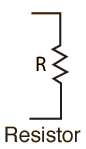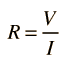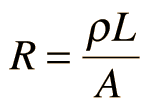# ResistanceThe electrical resistance of a circuit component or device is defined as the ratio of the voltage applied to the electric current which flows through it:If the resistance is constant over a considerable range of voltage, then Ohm's law, I = V/R, can be used to predict the behavior of the material. Although the definition above involves DC current and voltage, the same definition holds for the AC application of resistors.

Whether or not a material obeys Ohm's law, its resistance can be described in terms of its bulk resistivity. The resistivity, and thus the resistance, is temperature dependent. Over sizable ranges of temperature, this temperature dependence can be predicted from a temperature coefficient of resistance.

 Conductors and insulators Resistor combinations Non-ohmic resistance: the electric pickle
 AC behavior of resistor Common carbon resistors
Index

DC Circuits

 HyperPhysics*****Electricity and magnetism R Nave
Go Back

# Resistivity and Conductivity

The electrical resistance of a wire would be expected to be greater for a longer wire, less for a wire of larger cross sectional area, and would be expected to depend upon the material out of which the wire is made. Experimentally, the dependence upon these properties is a straightforward one for a wide range of conditions, and the resistance of a wire can be expressed asThe factor in the resistance which takes into account the nature of the material is the resistivity . Although it is temperature dependent, it can be used at a given temperature to calculate the resistance of a wire of given geometry.

It should be noted that it is being presumed that the current is uniform across the cross-section of the wire, which is true only for Direct Current. For Alternating Current there is the phenomenon of "skin effect" in which the current density is maximum at the maximum radius of the wire and drops for smaller radii within the wire. At radio frequencies, this becomes a major factor in design because the outer part of a wire or cable carries most of the current.

The inverse of resistivity is called conductivity. There are contexts where the use of conductivity is more convenient.

Electrical conductivity = σ = 1/ρ

 Calculation Table of resistivities Common wire gauges
 Microscopic view of resistivity
Index

 HyperPhysics*****Electricity and magnetism R Nave
Go Back

# Resistor Combinations

The combination rules for any number of resistors in series or parallel can be derived with the use of Ohm's Law, the voltage law, and the current law.Comparison example
Index

DC Circuits

 HyperPhysics*****Electricity and magnetism R Nave
Go Back

# Resistivity Calculation

The electrical resistance of a wire would be expected to be greater for a longer wire, less for a wire of larger cross sectional area, and would be expected to depend upon the material out of which the wire is made (resistivity). Experimentally, the dependence upon these properties is a straightforward one for a wide range of conditions, and the resistance of a wire can be expressed asResistance = resistivity x length/area
For a wire of length L = m = ft
and area A = cm2
corresponding to radius r = cm
and diameter inches for common wire gauge comparison
with resistivity = ρ = x 10^ ohm meters
will have resistance R = ohms.

Enter data and then click on the quantity you wish to calculate in the active formula above. Unspecified parameters will default to values typical of 10 meters of #12 copper wire. Upon changes, the values will not be forced to be consistent until you click on the quantity you wish to calculate.

Commonly used U.S. wire gauges
for copper wire.
 AWG Diameter(inches) Typical use 10 0.1019 Electric range 12 0.0808 Household circuit 14 0.0640 Switch leads
 Standard wire gauges
Resistivities of some metals
in ohm-m(x 10-8) at 20°C.
 Aluminum 2.65 Gold 2.24 Copper 1.724 Silver 1.59 Iron 9.71 Platinum 10.6 Nichrome 100 Tungsten 5.65
 Table of resistivities

The factor in the resistance which takes into account the nature of the material is the resistivity. Although it is temperature dependent, it can be used at a given temperature to calculate the resistance of a wire of given geometry.

 Discussion Table of resistivities Common wire gauges
Index

 HyperPhysics*****Electricity and magnetism R Nave
Go Back